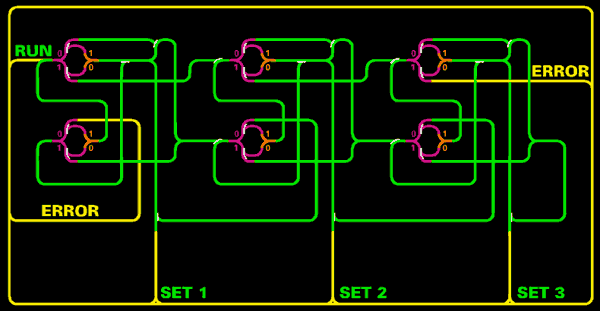Abstract Pictures Schematics Backgroundinfo (pdf) ContactThere are three types of points in the layout. - The distributors are orange; - The lazy points look the same but appear in different colors; - And the most common point is the sprung point, which is drawn with a clear preference for the route of the train. In order to prep the layout for input, all distributors and lazy points have to be set to 0. This is done, by pressing the RESET-Button. Now, the tape is set to 000. To set the input to the tape, press the red SET-Buttons. So to set up the input 1+1 on the three-block layout, you need to send the train to the outer two SET lines: 1 0 1. Then press SET1, leave SET2 untouched and press SET3. These Buttons control the three points on the yellow ringline. Afterwards the green RUN-Button lets the train first write the INPUT-value into each read/write head. It then gets directed into the SET1 read/writehead and comes back out on that line. This has effectively set the digit stored in that block on the "tape" to 1. It passes the SET2 point, moves into SET 3 and sets this value to 1. Now the Input 1 0 1 is set - visible through the I/0-lamps. The next station is the START point – Once the locomotive enters the system from that point the calculating starts. Now watch the train as it leaves again and finds a rest at the start point. The altered state, visible on the lamps, is the result. In this example 1 1 0, actually means 2 in the notation of this apparatus.   CALCULATING PROCEDURE To set up the train set for a calculation it needs to be re-set. By pressing the yellow RESET Button all points get set to the value "0". This is visible through the three 1/0-lightsigns. Now one can re-set the machine, by pressing the red SET-Buttons. After this the locomotive is ready to calculate and it gets started with the green RUN-Button. First the requested value gets written into the read/write-head (pink and orange) and then the train enters the system at the start-rail and gets directed through the system as the points have been pre-set and eventually leaves after a while. The altered state is the result. The following operations can be calculated:               Input      Output     0+0          000         000       = 0 0+1          010         100       = 1 1+0          100         100       = 1 1+1          101         110       = 2 0+2          011         110       = 2 2+0          110         110       = 2